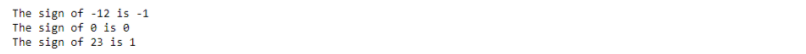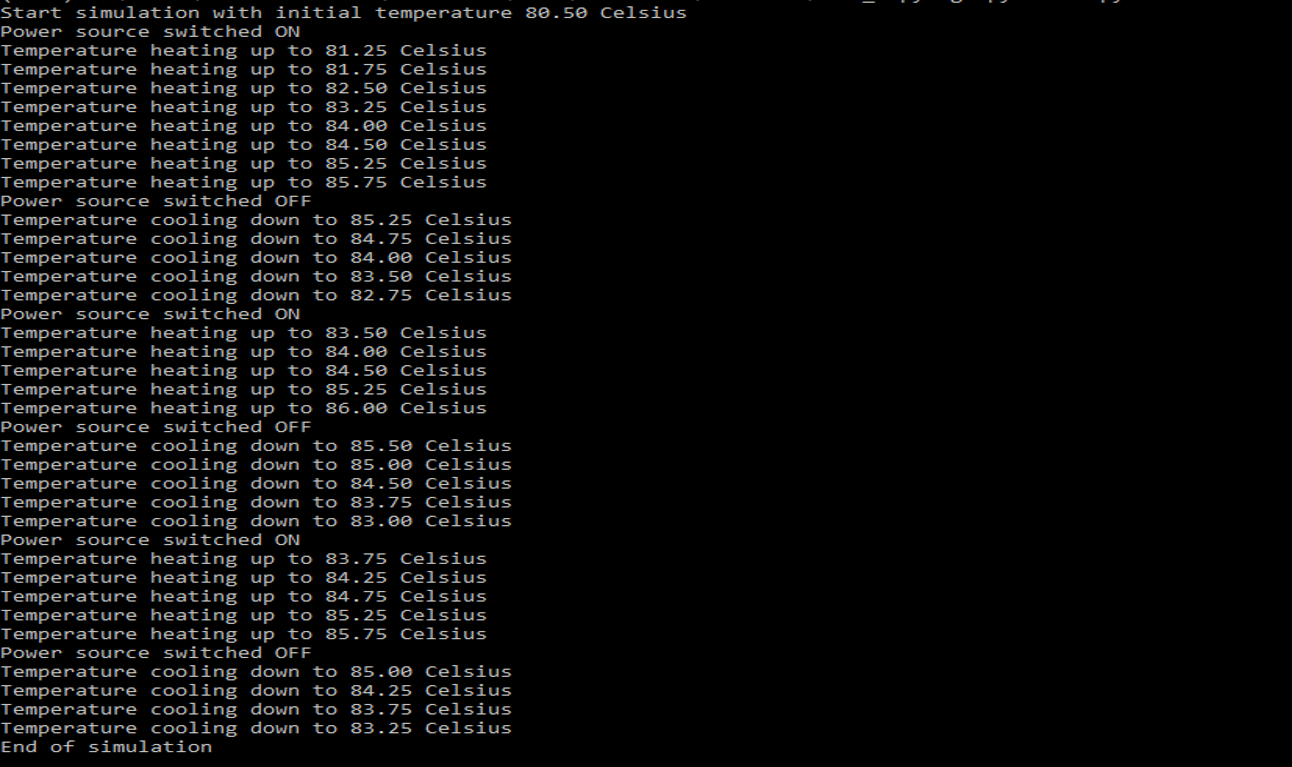## Python math.copysign

##### Aniekan

Python offers a wide array of mathematical functions with its math module. One of them, Python math.copysign function, allows us to copy the sign of one number to another number. In this article, we will begin by explaining the basics of Python math.copysign, and show some usage examples, before diving into two possible applications of the function. So, let us begin.

## Basics of Python math.copysign

The function Python math.copysign takes two parameters x and y, then returns a new value having the absolute value of x and the sign of y. This function proves to be helpful if we ever need to make sure that one number has the sign of another.

The simple code snippet below shows how to call Python math.copysign:

import math

x = 3
y = -5

new_number = math.copysign(x, y) # new_number will contain -3


In the above code, when the call to math.copysign returns, the value of -3 will be returned and stored in the variable new_number.

The variable x is the number whose magnitude will be used for the returned value of math.copysign.

The variable y is the number whose sign will be used for the final returned value of the function. Let us dive into more examples of how to make use of the math.copysign function in Python.

## Examples of using math.copysign

The first example is shown in the code snippet below.

In the example, we want to copy the sign of a positive number.

import math

x = 2
y = -2
z = -7

num1 = math.copysign(x, z) # num1 will contain -2
num2 = math.copysign(y, z) # num2 will contain -2

In our code, we want to copy the sign of z (which is negative, since z stores the value -7).

First, we combine the negative sign from z with the magnitude (or absolute value) of x. math.copysign will return -2 . In the second call to math.copysign, we want to combine the magnitude of y with the negative sign from z. Since y already stores a negative value, and z is also negative, the function simply returns the value of y unchanged.

The second example is similar, but we want to copy a positive sign to the magnitude of another number.

import math

x = 5
y = -5
z = 10

num1 = math.copysign(x, z) # num1 will contain 5
num2 = math.copysign(y, z) # num2 will contain 5

Both calls to math.copysign return 5 since we are copying the sign of a positive number 10 (stored in the variable z). The first call is actually redundant since the signs of x and z are already the same.

In summary, the behavior of math.copysign is as follows:

math.copysign(x,y) = abs(x)\times sgn(y)

where sgn(y) is the sign/signum function which returns the sign of y.

In the next section, we will explore how to use math.copysign to define the signum function, since Python does not define any in its built-in libraries.

## Implementing sgn(x) (signum function)

The signum function is a mathematical function that is defined as follows:

sgn(x) = \begin{cases} 1 & x>0 \\ 0 & x=0 \\ -1 & x<0\end{cases}

The numerical values returned are used to represent the sign of the input x. We can define our own version of the signum function by making use of math.copysign. The definition is as follows:

sgn(x) = \begin{cases} 0 & x=0 \\ copysign(1, x) & otherwise\end{cases}

Our signum function makes use of math.copysign when the value of x is not equal to 0. The copysign function returns +1 if x > 0 or -1 if x < 0.

The code example below shows a Python definition for our very own signum function.

import math

def sgn(x):
if x==0:
return 0

return int(math.copysign(1, x))

x = -12
y = 0
z = 23

print(f"The sign of {x} is {sgn(x)}")
print(f"The sign of {y} is {sgn(y)}")
print(f"The sign of {z} is {sgn(z)}")

Our code snippet also contains three different calls to our sgn function, checking for the signs of x, y, and z. The first one check . The output is as follows:As expected, -12 has a negative sign, while 23 has a positive sign (represented by the int values -1 and 1 respectively). The special case 0 is neutral, and has a sign represented by 0.

## Simulating a Thermostat using math.copysign

We want to explore how to make use of math.copysign to simulate a Thermostat.

Specifically, we will make use of math.copysign to regulate the temperature.

A Thermostat is a temperature-regulating device used to control the temperature of a space.

The way a simple thermostat system works is that when the temperature rises above a setpoint (or target temperature), the thermostat switches off the heat source, but switches it back on when the temperature falls back below the setpoint.

In this way, the thermostat maintains the temperature of the system around the target temperature.

The code below demonstrates our Temperature regulation simulation.

We have defined two classes: HeatSource to represent a heating source that can be powered ON or OFF, and the Thermostat class to monitor the current temperature of the HeatSourceso that the temperature falls within a particular range.

The source code is shown below:

from math import copysign
import random
import time

class HeatSource:
def __init__(self):
self.__isPoweredON = False
self.__currentTemp = 80.5

@property
def currentTemp(self):
return self.__currentTemp

@property
def isPoweredON(self):
return self.__isPoweredON

def powerON(self):
self.__isPoweredON = True

def powerOFF(self):
self.__isPoweredON = False

def updateTemp(self):
step = random.choice([0.50, 0.75])
if self.__isPoweredON:
self.__currentTemp += step
print(f"Temperature heating up to {self.__currentTemp:.2f} Celsius")

else:
self.__currentTemp -= step
print(f"Temperature cooling down to {self.__currentTemp:.2f} Celsius")

class Thermostat:
def __init__(self, hs):
self.__heat_source = hs
self.__low_threshold = 83.5
self.__high_threshold = 85.5

def checkTemp(self):
low_temp_diff = self.__heat_source.currentTemp - self.__low_threshold
high_temp_diff = self.__heat_source.currentTemp - self.__high_threshold
if int(copysign(1, low_temp_diff))==-1 and not self.__heat_source.isPoweredON:
self.__heat_source.powerON()
print("Power source switched ON")

elif int(copysign(1, high_temp_diff))==1 and self.__heat_source.isPoweredON:
self.__heat_source.powerOFF()
print("Power source switched OFF")

self.__heat_source.updateTemp()

def main():
hs = HeatSource()
th = Thermostat(hs)

print(f"Start simulation with initial temperature {hs.currentTemp:.2f} Celsius")

while True:
try:
th.checkTemp()
time.sleep(1.0)

except KeyboardInterrupt:
print("End of simulation")
break

if __name__=="__main__":
main()

We see how math.copysign was used within the checkTemp method of Thermostatto determine when to turn the heat source ON or OFF. checkTempdoes the regulation as follows:

• Check for Low Temperature Threshold: checkTemp checks if the temperature is below the lower temperature threshold by checking the sign of the difference between the current temperature of the heat source and the low threshold. If the difference is negative, it switches on the heat source.
• Check for High Temperature Threshold: In order to switch off the heat source, checkTemp calculates the difference between the current temperature of the heat source and the high threshold. If the difference is positive, it switches on the heat source so that the system heats up.

When our script is run, we get something similar to the following:Our heat source first starts with a temperature of 80.50\degree C.

The thermostat allows the heat source to heat up to the high-temperature threshold of 85.50\degree C, before switching off the heat source to let it cool down.

When the heat source cools below the low threshold of 83.50\degree C, our thermostat switches on the heat source again, and the process repeats. We added the ability to stop our simulation by pressing the key combination CTRL+C.

In conclusion, we have seen how helpful math.copysign can be in allowing us to make decisions based on the sign of numbers.

We have also shown how to use it to create our own implementation of the signum function in Python.

We have also used the math.copysign function to implement a temperature regulation system.

If you liked this tutorial, please feel free to check out our other tutorials on different topics relating to Python programming.

Thanks, and Happy coding!!!